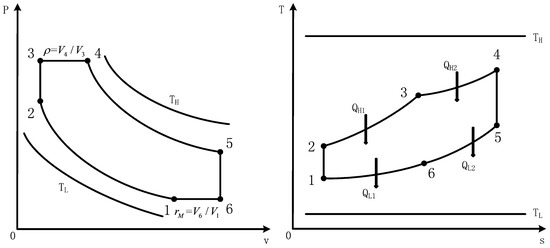Next Article in Journal
Statistics of Correlations and Fluctuations in a Stochastic Model of Wealth Exchange
Previous Article in Journal
Uncertainty Evaluation in Multistage Assembly Process Based on Enhanced OOPN
Open AccessArticle

Thermodynamic Optimization for an Endoreversible Dual-Miller Cycle (DMC) with Finite Speed of Piston

1
Institute of Thermal Science and Power Engineering, Naval University of Engineering, Wuhan 430033, China
2
Military Key Laboratory for Naval Ship Power Engineering, Naval University of Engineering, Wuhan 430033, China
3
College of Power Engineering, Naval University of Engineering, Wuhan 430033, China
*
Author to whom correspondence should be addressed.
Entropy 2018, 20(3), 165; https://doi.org/10.3390/e20030165
Received: 16 December 2017 / Revised: 28 February 2018 / Accepted: 1 March 2018 / Published: 5 March 2018
(This article belongs to the Section Thermodynamics)
Power output ( $P$ ), thermal efficiency ( $η$ ) and ecological function ( $E$ ) characteristics of an endoreversible Dual-Miller cycle (DMC) with finite speed of the piston and finite rate of heat transfer are investigated by applying finite time thermodynamic (FTT) theory. The parameter expressions of the non-dimensional power output ( $P ¯$ ), $η$ and non-dimensional ecological function ( $E ¯$ ) are derived. The relationships between $P ¯$ and cut-off ratio ( $ρ$ ), between $P ¯$ and $η$ , as well as between $E ¯$ and $ρ$ are demonstrated. The influences of $ρ$ and piston speeds in different processes on $P ¯$ , $η$ and $E ¯$ are investigated. The results show that $P ¯$ and $E ¯$ first increase and then start to decrease with increasing $ρ$ . The optimal cut-off ratio $ρ o p t$ will increase if piston speeds increase in heat addition processes and heat rejection processes. As piston speeds in different processes increase, the maximum values of $P ¯$ and $E ¯$ increase. The results include the performance characteristics of various simplified cycles of DMC, such as Otto cycle, Diesel cycle, Dual cycle, Otto-Atkinson cycle, Diesel-Atkinson cycle, Dual-Atkinson cycle, Otto-Miller cycle and Diesel-Miller cycle. Comparing performance characteristics of the DMC with different optimization objectives, when choosing $E ¯$ as optimization objective, $η$ improves 26.4% compared to choosing $P ¯$ as optimization objective, while $P ¯$ improves 74.3% compared to choosing $η$ as optimization objective. Thus, optimizing $E$ is the best compromise between optimizing $P$ and optimizing $η$ . The results obtained can provide theoretical guidance to design practical DMC engines. View Full-Text
Show FiguresFigure 1

MDPI and ACS Style

Wu, Z.; Chen, L.; Feng, H. Thermodynamic Optimization for an Endoreversible Dual-Miller Cycle (DMC) with Finite Speed of Piston. Entropy 2018, 20, 165.

Show more citation formats Show less citations formats
Note that from the first issue of 2016, MDPI journals use article numbers instead of page numbers. See further details here.

1